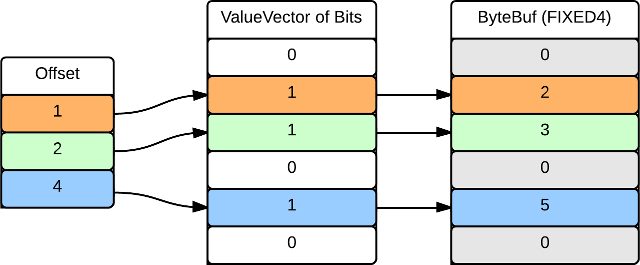# Value Vectors

This document defines the data structures required for passing sequences of columnar data between Operators.

## Goals

### Support Operators Written in Multiple Language

ValueVectors should support operators written in C/C++/Assembly. To support this, the underlying ByteBuffer will not require modification when passed through the JNI interface. The ValueVector will be considered immutable once constructed. Endianness has not yet been considered.

### Access

Reading a random element from a ValueVector must be a constant time operation. To accomodate, elements are identified by their offset from the start of the buffer. Repeated, nullable and variable width ValueVectors utilize in an additional fixed width value vector to index each element. Write access is not supported once the ValueVector has been constructed.

### Efficient Subsets of Value Vectors

When an operator returns a subset of values from a ValueVector, it should reuse the original ValueVector. To accomplish this, a level of indirection is introduced to skip over certain values in the vector. This level of indirection is a sequence of offsets which reference an offset in the original ValueVector and the count of subsequent values which are to be included in the subset.

### Pooled Allocation

ValueVectors utilize one or more buffers under the covers. These buffers will be drawn from a pool. Value vectors are themselves created and destroyed as a schema changes during the course of record iteration.

### Homogenous Value Types

Each value in a Value Vector is of the same type. The Record Batch implementation is responsible for creating a new Value Vector any time there is a change in schema.

## Definitions

Data Types

The canonical source for value type definitions is the Drill Datatypes document. The individual types are listed under the ‘Basic Data Types’ tab, while the value vector types can be found under the ‘Value Vectors’ tab.

Operators

An operator is responsible for transforming a stream of fields. It operates on Record Batches or constant values.

Record Batch

A set of field values for some range of records. The batch may be composed of Value Vectors, in which case each batch consists of exactly one schema.

Value Vector

The value vector is comprised of one or more contiguous buffers; one which stores a sequence of values, and zero or more which store any metadata associated with the ValueVector.

## Data Structure

A ValueVector stores values in a ByteBuf, which is a contiguous region of memory. Additional levels of indirection are used to support variable value widths, nullable values, repeated values and selection vectors. These levels of indirection are primarily lookup tables which consist of one or more fixed width ValueVectors which may be combined (e.g. for nullable, variable width values). A fixed width ValueVector of non-nullable, non-repeatable values does not require an indirect lookup; elements can be accessed directly by multiplying position by stride.

Fixed Width Values

Fixed width ValueVectors simply contain a packed sequence of values. Random access is supported by accessing element n at ByteBuf + Index * Stride, where Index is 0-based. The following illustrates the underlying buffer of INT4 values [1 .. 6]: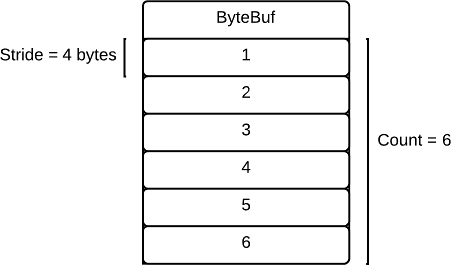Nullable Values

Nullable values are represented by a vector of bit values. Each bit in the vector corresponds to an element in the ValueVector. If the bit is not set, the value is NULL. Otherwise the value is retrieved from the underlying buffer. The following illustrates a NullableValueVector of INT4 values 2, 3 and 6: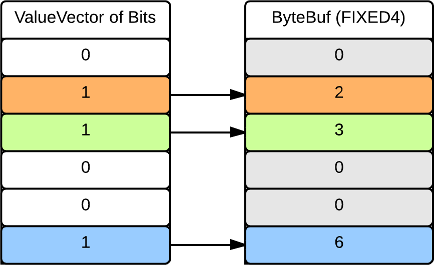### Repeated Values

A repeated ValueVector is used for elements which can contain multiple values (e.g. a JSON array). A table of offset and count pairs is used to represent each repeated element in the ValueVector. A count of zero means the element has no values (note the offset field is unused in this case). The following illustrates three fields; one with two values, one with no values, and one with a single value: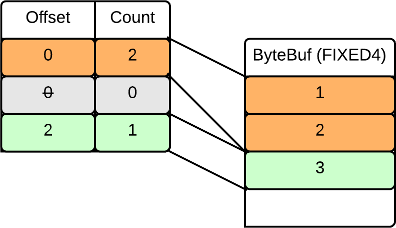ValueVector Representation of the equivalent JSON:

x:[1, 2]

x:[ ]

x:

Variable Width Values

Variable width values are stored contiguously in a ByteBuf. Each element is represented by an entry in a fixed width ValueVector of offsets. The length of an entry is deduced by subtracting the offset of the following field. Because of this, the offset table will always contain one more entry than total elements, with the last entry pointing to the end of the buffer.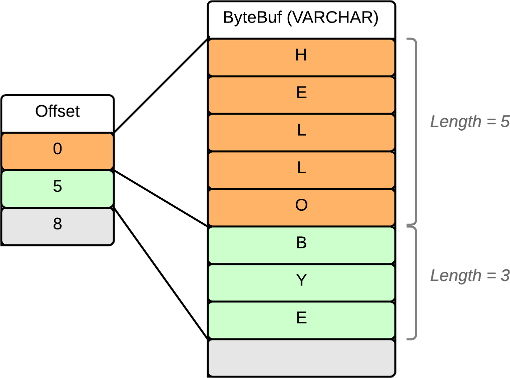Repeated Map Vectors

A repeated map vector contains one or more maps (akin to an array of objects in JSON). The values of each field in the map are stored contiguously within a ByteBuf. To access a specific record, a lookup table of count and offset pairs is used. This lookup table points to the first repeated field in each column, while the count indicates the maximum number of elements for the column. The following example illustrates a RepeatedMap with two records; one with two objects, and one with a single object: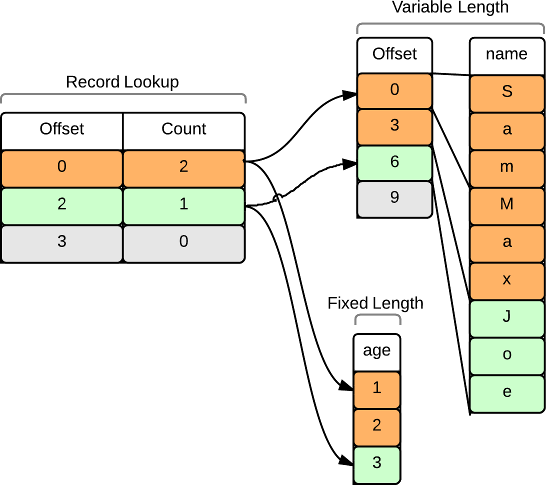ValueVector representation of the equivalent JSON:

x: [ {name:’Sam’, age:1}, {name:’Max’, age:2} ]

x: [ {name:’Joe’, age:3} ]

Selection Vectors

A Selection Vector represents a subset of a ValueVector. It is implemented with a list of offsets which identify each element in the ValueVector to be included in the SelectionVector. In the case of a fixed width ValueVector, the offsets reference the underlying ByteBuf. In the case of a nullable, repeated or variable width ValueVector, the offset references the corresponding lookup table. The following illustrates a SelectionVector of INT4 (fixed width) values 2, 3 and 5 from the original vector of [1 .. 6]: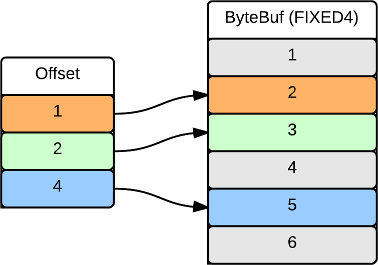The following illustrates the same ValueVector with nullable fields: